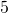# Factor Trinomial

On this page we practise factoring a trinomial of the form# Why factor a trinomial?

Factoring is a very nice skill to have when we want to solve quadratic equations (grade 11 math). By factoring, we can arrive at solutions quickly which can be rather satisfying. Factoring also allows us to use a different form of the same expression and find out more about it. It can be helpful when proving results in other areas of mathematics, such as vector geometry and calculus.

However, when it comes to solving quadratics, factoring is only helpful when it can be done reasonably quickly. Otherwise, we have the ‘quadratic formula’ to help us out.

# The puzzle

The first skill required to factor a trinomial is to be able to expand the brackets. Each time you factor, you should expand the brackets (at least mentally if not on paper) to make sure your factored expression is equivalent to the expression you began with.

The second skill required is to be able to solve the numerical puzzle – that is, to spot a number pair that multiplies to the constant and adds to the coefficient of. On this first applet, you are to think of the two integers that add to the value given in the first box, and the multiply to the value in the second box. Hit ‘check’ when you think you know. Keep going until you are getting a whole bunch correct!

# Factor a Trinomial

Video one:  three examples from the applet below

Example:We need to find numbersandsuch thatA little thought or writing on paper leads us to the valuesandbecauseand.

Therefore we haveCheck this answer by expanding the brackets with FOIL or otherwise to confirm that the expression on the left hand side is equivalent to the expression on the right hand side.

Now we are ready to practice the factoring level 1, where. Note: all the trinomials given here factor nicely with integers. This is not always the case – chances are pretty high that if you make up a random trinomial, it won’t factor so nicely.